• CNN: 1\ Siamoid 2\ Relu + Softplus 图片来源： http://ufldl.stanford.edu/tutorial/supervised/MultiLayerNeuralNetworks/ https://www.cnblogs.com/eniac1946/p/7878128.html 转载于:...

CNN:
1\ Siamoid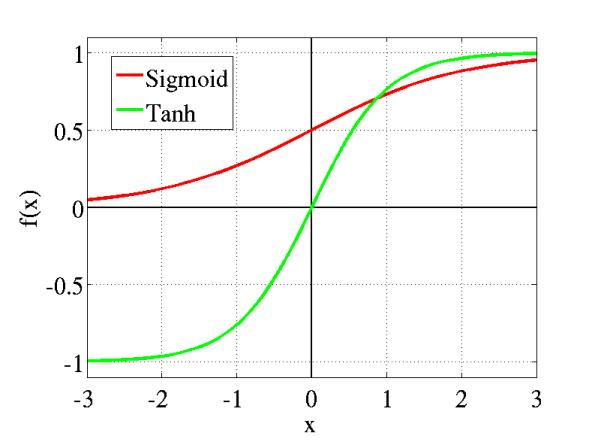2\ Relu + Softplus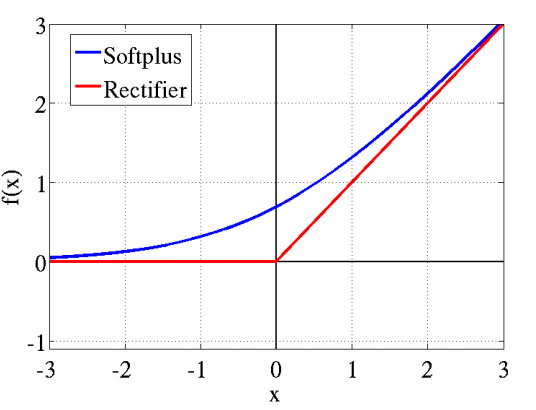图片来源：
http://ufldl.stanford.edu/tutorial/supervised/MultiLayerNeuralNetworks/
https://www.cnblogs.com/eniac1946/p/7878128.html

转载于:https://www.cnblogs.com/xiaoniu-666/p/10554600.html
展开全文• CNN - 激活函数 首先明白函数的作用：增加模型的非线性表达能力 感谢: 蒋竺波：CNN入门讲解：什么是激活函数（Activation Function） 前提知识复习： 参考知乎：什么是「齐次」，「非齐次」，「线性」，「非线性」...
CNN - 激活函数
首先明白函数的作用：增加模型的非线性表达能力
感谢: 蒋竺波：CNN入门讲解：什么是激活函数（Activation Function）

前提知识复习：
参考知乎：什么是「齐次」，「非齐次」，「线性」，「非线性」？ - 知乎
可加性：$f(x_1+x_2) = f(x_1) + f(x_2)$
齐次性：$f(kx_1) = kf(x_1)$ or $f(k^\alpha x_1) = \alpha kf(x_1)$
线性：从字面上看“线性”就是“具有线的特性”，这里的“线”指的是直线
线性：$f(ax_1+bx_2) = af(x_1)+bf(x_2)$，可加且齐次，eg:旋转、缩放
线性 具有可加性和齐次性
故：任意的线性函数都可以等价为一个单一线性函数
而，线性函数能把平面分为2部分，有分类的效果。

线性 设法解决 非线性 问题
现有一次线性函数: $Y=W*X+B$
No1：那么就可以用线性分割非线性($y=ax^2+b$)， 来解决非线性问题；
No2：用线性分割进行分类，eg：$分类结果=\begin{cases} 0 & y<0 \\ 1 & y>0\end{cases}$；
No3，error，没懂；
No4，反向传播，没懂
线性与激活函数的关系

函数是线性的，导数是常数
梯度与 $x$ 无关(方向导数与梯度)

激活函数(Activation Function)
1. 阶跃函数
二分类 非线性 激活函数
$f(x) = \begin{cases}0&y<0\\1&y>0\end{cases}$
2. sigmoid函数
$\sigma(x)=\frac{1}{1+e^{-x}}$
3. tanh函数
$\tan h(x)$
对比sigmoid，这个可以输出负值
4. ReLU函数
$\max(0, x)$
应用场景：输入值不能是非负数，对于图片格式，ReLU就挺常用的
参考1：数学公式输入
参考2：分段函数的大括号输入


展开全文• 1、什么是激活函数 激活函数(Activation functions)对于人工神经网络 模型去学习、理解非常复杂和非线性的函数来说具有十分重要的作用。它们将非线性特性引入到我们的网络中。如下图，在神经元中，输入的 inputs ...
目录

1、什么是激活函数

2、为什么要使用激活函数？

3、为什么激活函数需要非线性函数？

4、常用的激活函数

sigmoid 激活函数

tanh激活函数

Relu激活函数

Leaky ReLU函数（PReLU）

ELU激活函数

Mish激活函数

Swish 激活函数

SiLU激活函数

1、什么是激活函数

激活函数(Activation functions)对于人工神经网络 模型去学习、理解非常复杂和非线性的函数来说具有十分重要的作用。它们将非线性特性引入到我们的网络中。如下图，在神经元中，输入的 inputs 通过加权，求和后，还被作用了一个函数，这个函数就是激活函数。引入激活函数是为了增加神经网络模型的非线性。没有激活函数的每层都相当于矩阵相乘。就算你叠加了若干层之后，无非还是个矩阵相乘罢了。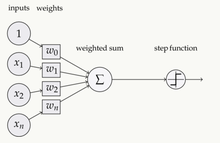2、为什么要使用激活函数？

激活函数对模型学习、理解非常复杂和非线性的函数具有重要作用。
激活函数可以引入非线性因素。如果不使用激活函数，则输出信号仅是一个简单的线性函数。线性函数一个一级多项式，线性方程的复杂度有限，从数据中学习复杂函数映射的能力很小。没有激活函数，神经网络将无法学习和模拟其他复杂类型的数据，例如图像、视频、音频、语音等。
激活函数可以把当前特征空间通过一定的线性映射转换到另一个空间，让数据能够更好的被分类。
3、为什么激活函数需要非线性函数？

假若网络中全部是线性部件，那么线性的组合还是线性，与单独一个线性分类器无异。这样就做不到用非线性来逼近任意函数。
使用非线性激活函数 ，以便使网络更加强大，增加它的能力，使它可以学习复杂的事物，复杂的表单数据，以及表示输入输出之间非线性的复杂的任意函数映射。使用非线性激活函数，能够从输入输出之间生成非线性映射。
4、常用的激活函数

sigmoid 激活函数

函数的定义为：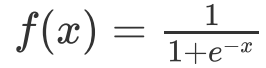其值域为 (0,1) 。函数图像如下：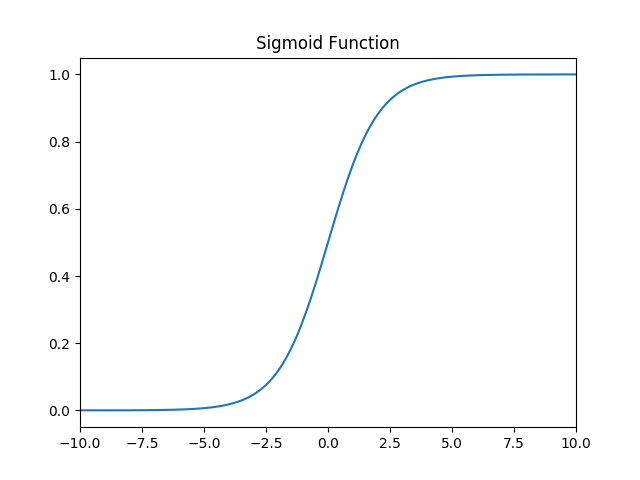特点：
它能够把输入的连续实值变换为0和1之间的输出，特别的，如果是非常大的负数，那么输出就是0；如果是非常大的正数，输出就是1.
缺点：
sigmoid函数曾经被使用的很多，不过近年来，用它的人越来越少了。主要是因为它固有的一些 缺点。
缺点1：在深度神经网络中梯度反向传递时导致梯度爆炸和梯度消失，其中梯度爆炸发生的概率非常小，而梯度消失发生的概率比较大。首先来看Sigmoid函数的导数，如下图所示：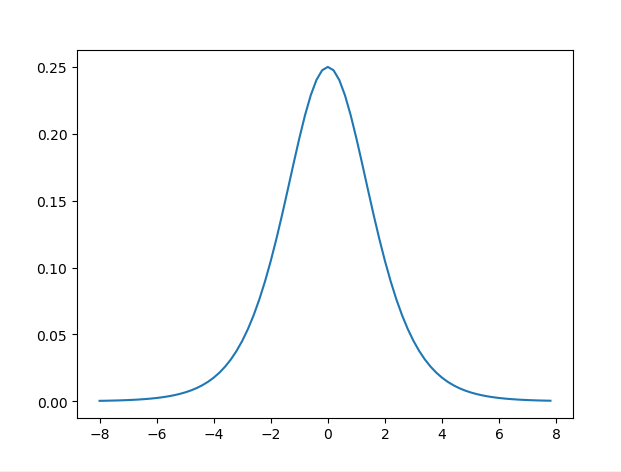缺点2：不是以0为对称轴（这点在tahn函数有所改善）

sigmoid函数及其导数的实现

import numpy as np
import matplotlib.pyplot as plt

#解决中文显示问题
plt.rcParams['font.sans-serif']=['SimHei']
plt.rcParams['axes.unicode_minus'] = False
def d_sigmoid(x):
y = 1 / (1 + np.exp(-x))
dy=y*(1-y)
return dy

def sigmoid(x):
y = 1 / (1 + np.exp(-x))
return y

def plot_sigmoid():
# param:起点，终点，间距
x = np.arange(-8, 8, 0.2)
plt.subplot(1, 2, 1)
plt.title('sigmoid')  # 第一幅图片标题
y = sigmoid(x)
plt.plot(x, y)
plt.subplot(1, 2, 2)
y = d_sigmoid(x)
plt.plot(x, y)
plt.title('sigmoid导数')
plt.show()

if __name__ == '__main__':
plot_sigmoid()


tanh激活函数

函数的定义为：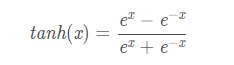其值域为 (-1,1) 。函数图像如下：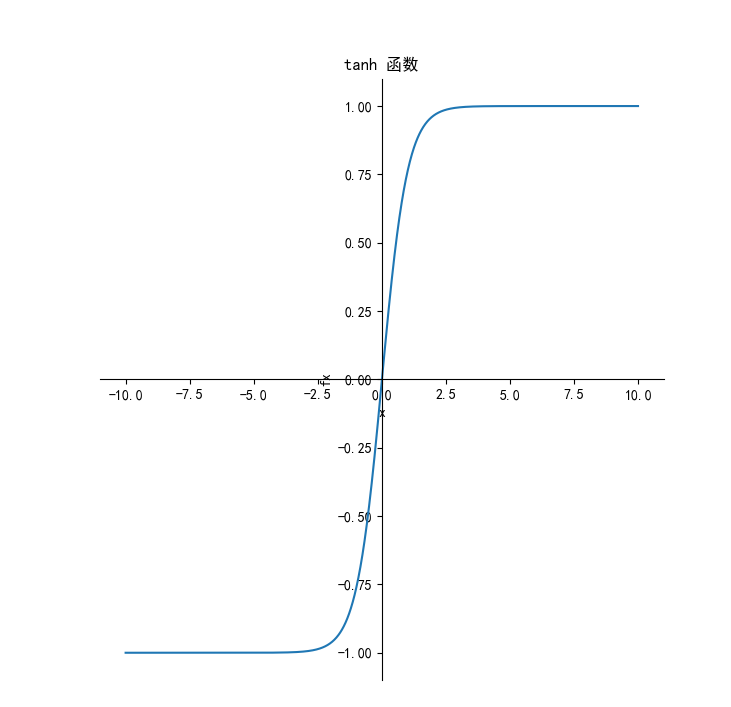导数：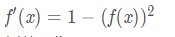函数图像如下：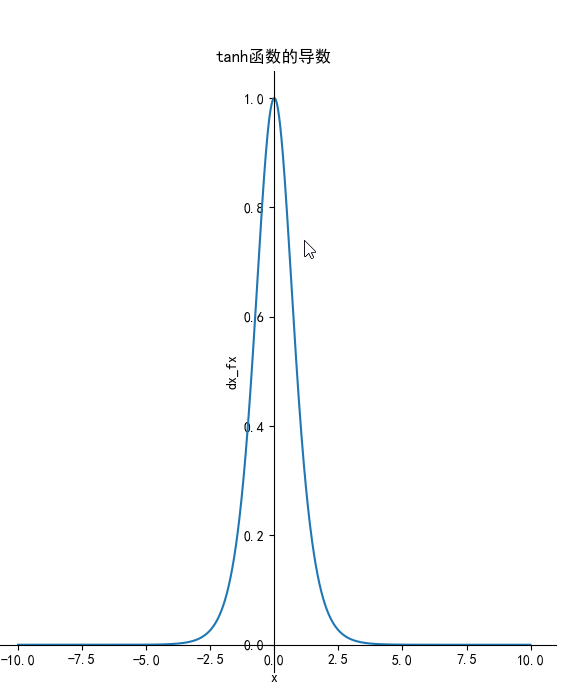tanh读作Hyperbolic Tangent，它解决了Sigmoid函数的不是zero-centered输出问题，然而，梯度消失（gradient vanishing）的问题和幂运算的问题仍然存在。

优点和缺点

优点：
解决了Sigmoid的输出不关于零点对称的问题
也具有Sigmoid的优点平滑，容易求导

缺点：
激活函数运算量大（包含幂的运算
Tanh的导数图像虽然最大之变大，使得梯度消失的问题得到一定的缓解，但是不能根本解决这个问题

tanh函数及其代码实现：

from matplotlib import pyplot as plt
import numpy as np

# 解决中文显示问题
plt.rcParams['font.sans-serif'] = ['SimHei']
plt.rcParams['axes.unicode_minus'] = False
def tanh(x):
"""tanh函数"""
return ((np.exp(x) - np.exp(-x)) / (np.exp(x) + np.exp(-x)))

def dx_tanh(x):
"""tanh函数的导数"""
return 1 - tanh(x) * tanh(x)

if __name__ == '__main__':
x = np.arange(-10, 10, 0.01)
fx = tanh(x)
dx_fx = dx_tanh(x)
plt.subplot(1, 2, 1)
ax = plt.gca()  # 得到图像的Axes对象
ax.spines['right'].set_color('none')  # 将图像右边的轴设为透明
ax.spines['top'].set_color('none')  # 将图像上面的轴设为透明
ax.xaxis.set_ticks_position('bottom')  # 将x轴刻度设在下面的坐标轴上
ax.yaxis.set_ticks_position('left')  # 将y轴刻度设在左边的坐标轴上
ax.spines['bottom'].set_position(('data', 0))  # 将两个坐标轴的位置设在数据点原点
ax.spines['left'].set_position(('data', 0))
plt.title('tanh 函数')
plt.xlabel('x')
plt.ylabel('fx')
plt.plot(x, fx)
plt.subplot(1, 2, 2)
ax = plt.gca()  # 得到图像的Axes对象
ax.spines['right'].set_color('none')  # 将图像右边的轴设为透明
ax.spines['top'].set_color('none')  # 将图像上面的轴设为透明
ax.xaxis.set_ticks_position('bottom')  # 将x轴刻度设在下面的坐标轴上
ax.yaxis.set_ticks_position('left')  # 将y轴刻度设在左边的坐标轴上
ax.spines['bottom'].set_position(('data', 0))  # 将两个坐标轴的位置设在数据点原点
ax.spines['left'].set_position(('data', 0))
plt.title('tanh函数的导数')
plt.xlabel('x')
plt.ylabel('dx_fx')
plt.plot(x, dx_fx)
plt.show()


Relu激活函数

它保留了 step 函数的生物学启发（只有输入超出阈值时神经元才激活），不过当输入为正的时候，导数不为零，从而允许基于梯度的学习（尽管在 x=0 的时候，导数是未定义的）。使用这个函数能使计算变得很快，因为无论是函数还是其导数都不包含复杂的数学运算。然而，当输入为负值的时候，ReLU 的学习速度可能会变得很慢，甚至使神经元直接无效，因为此时输入小于零而梯度为零，从而其权重无法得到更新，在剩下的训练过程中会一直保持静默。函数的定义为：f(x)=max(0,x),值阈[0,] 。函数图像如下：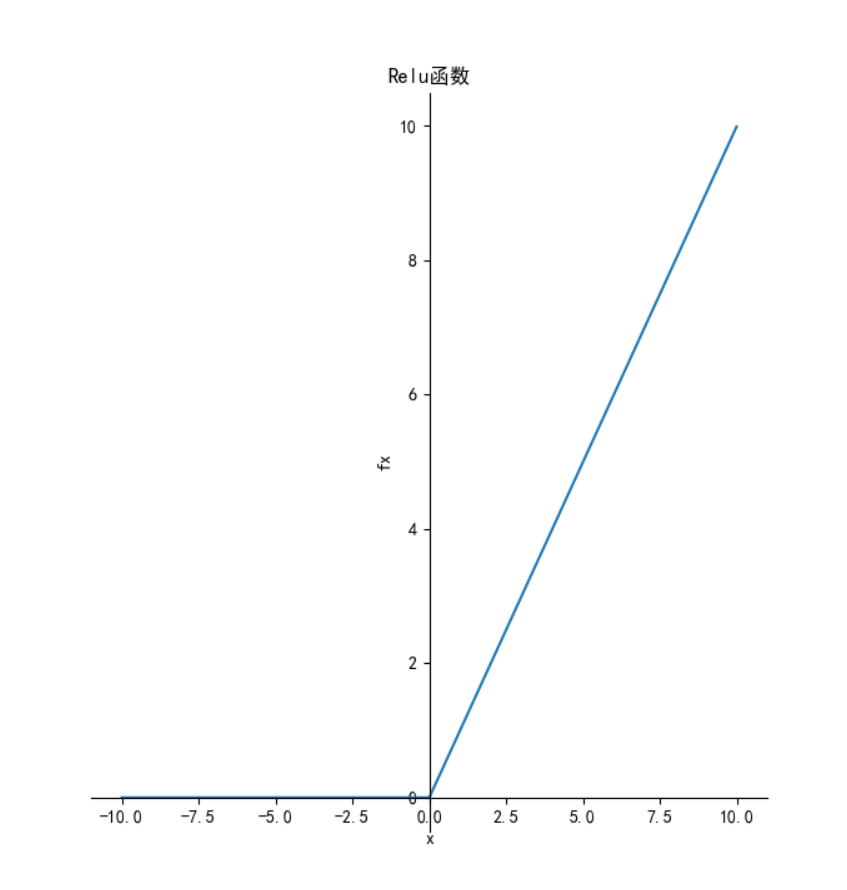导数：

函数图像如下：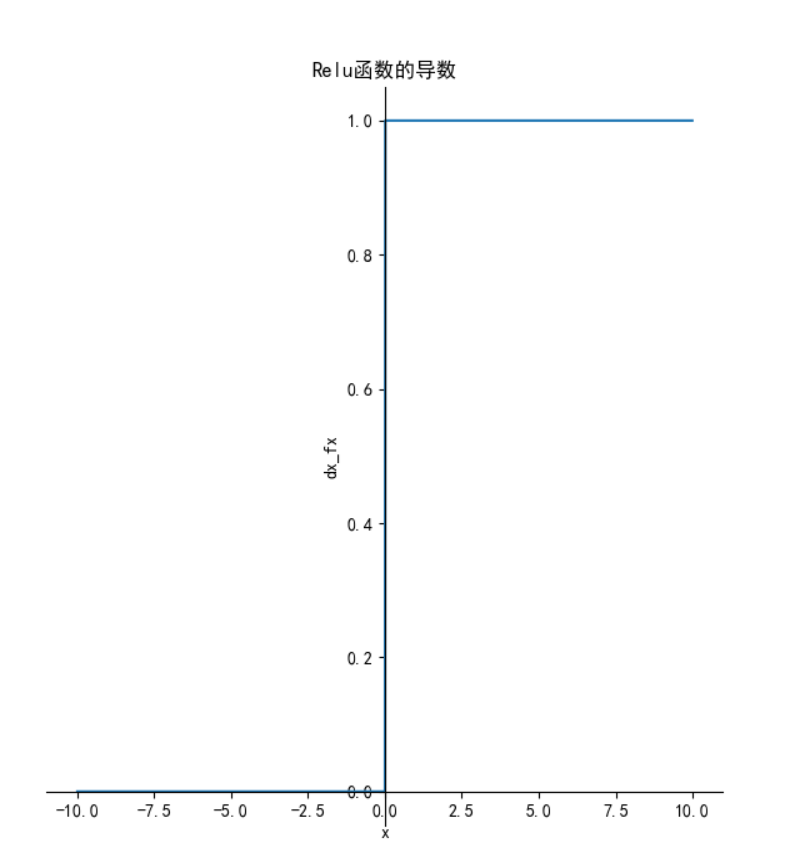优点：

1.相比起Sigmoid和tanh，ReLU在SGD中能够快速收敛，这是因为它线性（linear）、非饱和（non-saturating）的形式。

2.Sigmoid和tanh涉及了很多很expensive的操作（比如指数），ReLU可以更加简单的实现。

3.有效缓解了梯度消失的问题。

4.在没有无监督预训练的时候也能有较好的表现。

缺点：

ReLU的输出不是zero-centered
Dead ReLU Problem，指的是某些神经元可能永远不会被激活，导致相应的参数永远不能被更新。有两个主要原因可能导致这种情况产生: (1) 非常不幸的参数初始化，这种情况比较少见 (2) learning rate太高导致在训练过程中参数更新太大，不幸使网络进入这种状态。解决方法是可以采用Xavier初始化方法，以及避免将learning rate设置太大或使用adagrad等自动调节learning rate的算法。
尽管存在这两个问题，ReLU目前仍是最常用的activation function，在搭建人工神经网络的时候推荐优先尝试！

函数及导数代码：

from matplotlib import pyplot as plt
import numpy as np

# 解决中文显示问题
plt.rcParams['font.sans-serif'] = ['SimHei']
plt.rcParams['axes.unicode_minus'] = False
def relu(x):
"""relu函数"""
# temp = np.zeros_like(x)
# if_bigger_zero = (x > temp)
# return x * if_bigger_zero
return np.where(x<0,0,x)

def dx_relu(x):
"""relu函数的导数"""
# temp = np.zeros_like(x)
# if_bigger_equal_zero = (x >= temp)
# return if_bigger_equal_zero * np.ones_like(x)
return np.where(x < 0, 0, 1)
# ---------------------------------------------

if __name__ == '__main__':
x = np.arange(-10, 10, 0.01)
fx = relu(x)
dx_fx = dx_relu(x)
plt.subplot(1, 2, 1)
ax = plt.gca()  # 得到图像的Axes对象
ax.spines['right'].set_color('none')  # 将图像右边的轴设为透明
ax.spines['top'].set_color('none')  # 将图像上面的轴设为透明
ax.xaxis.set_ticks_position('bottom')  # 将x轴刻度设在下面的坐标轴上
ax.yaxis.set_ticks_position('left')  # 将y轴刻度设在左边的坐标轴上
ax.spines['bottom'].set_position(('data', 0))  # 将两个坐标轴的位置设在数据点原点
ax.spines['left'].set_position(('data', 0))
plt.title('Relu函数')
plt.xlabel('x')
plt.ylabel('fx')
plt.plot(x, fx)
plt.subplot(1, 2, 2)
ax = plt.gca()  # 得到图像的Axes对象
ax.spines['right'].set_color('none')  # 将图像右边的轴设为透明
ax.spines['top'].set_color('none')  # 将图像上面的轴设为透明
ax.xaxis.set_ticks_position('bottom')  # 将x轴刻度设在下面的坐标轴上
ax.yaxis.set_ticks_position('left')  # 将y轴刻度设在左边的坐标轴上
ax.spines['bottom'].set_position(('data', 0))  # 将两个坐标轴的位置设在数据点原点
ax.spines['left'].set_position(('data', 0))
plt.title('Relu函数的导数')
plt.xlabel('x')
plt.ylabel('dx_fx')
plt.plot(x, dx_fx)
plt.show()



Leaky ReLU函数（PReLU）

函数的定义为： 。函数图像如下：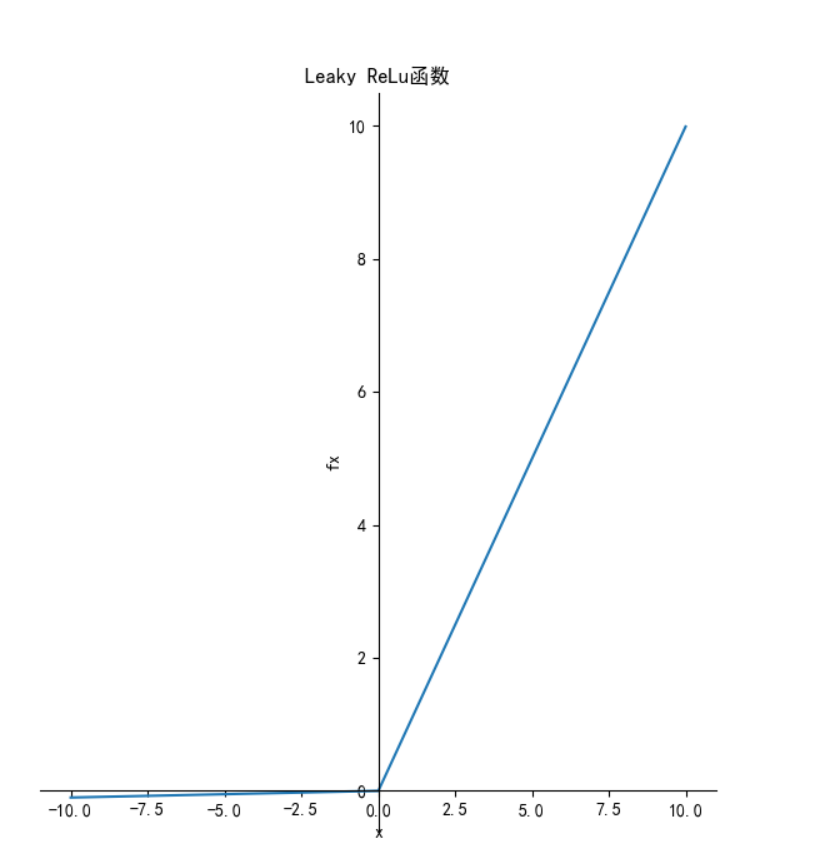导数：

函数图像如下：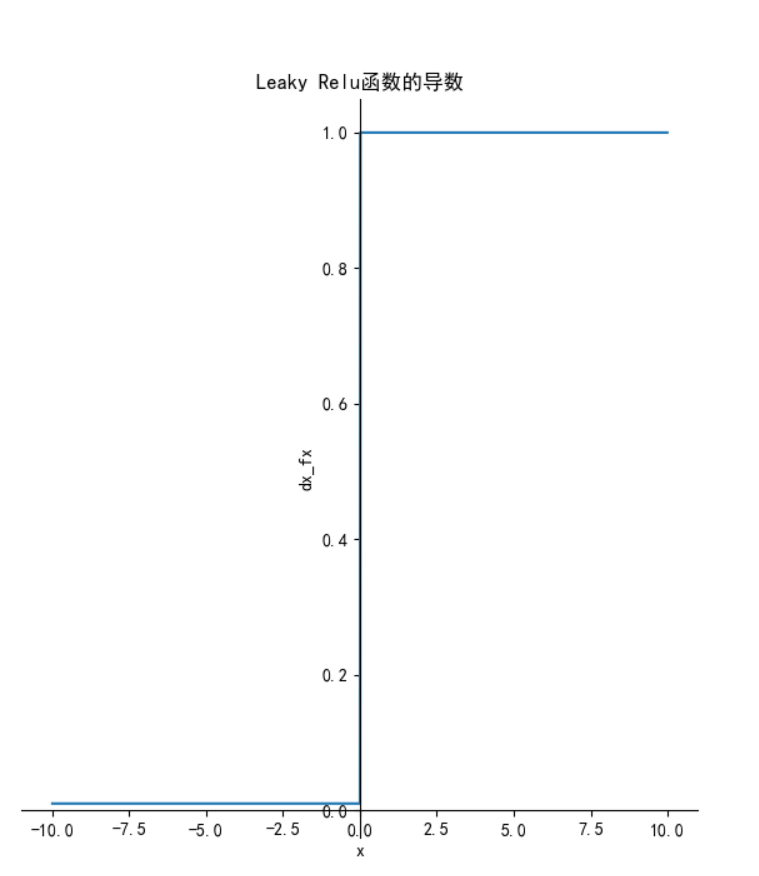特点：与 ReLu 相比 ，leak 给所有负值赋予一个非零斜率，  leak是一个很小的常数，这样保留了一些负轴的值，使得负轴的信息不会全部丢失。

函数及导数代码：

from matplotlib import pyplot as plt
import numpy as np

# 解决中文显示问题
plt.rcParams['font.sans-serif'] = ['SimHei']
plt.rcParams['axes.unicode_minus'] = False
def leaky_relu(x):
"""leaky relu函数"""
return np.where(x<0,0.01*x,x)

def dx_leaky_relu(x):
"""leaky relu函数的导数"""
return np.where(x < 0, 0.01, 1)

# ---------------------------------------------

if __name__ == '__main__':
x = np.arange(-10, 10, 0.01)
fx = leaky_relu(x)
dx_fx = dx_leaky_relu(x)
plt.subplot(1, 2, 1)
ax = plt.gca()  # 得到图像的Axes对象
ax.spines['right'].set_color('none')  # 将图像右边的轴设为透明
ax.spines['top'].set_color('none')  # 将图像上面的轴设为透明
ax.xaxis.set_ticks_position('bottom')  # 将x轴刻度设在下面的坐标轴上
ax.yaxis.set_ticks_position('left')  # 将y轴刻度设在左边的坐标轴上
ax.spines['bottom'].set_position(('data', 0))  # 将两个坐标轴的位置设在数据点原点
ax.spines['left'].set_position(('data', 0))
plt.title('Leaky ReLu函数')
plt.xlabel('x')
plt.ylabel('fx')
plt.plot(x, fx)
plt.subplot(1, 2, 2)
ax = plt.gca()  # 得到图像的Axes对象
ax.spines['right'].set_color('none')  # 将图像右边的轴设为透明
ax.spines['top'].set_color('none')  # 将图像上面的轴设为透明
ax.xaxis.set_ticks_position('bottom')  # 将x轴刻度设在下面的坐标轴上
ax.yaxis.set_ticks_position('left')  # 将y轴刻度设在左边的坐标轴上
ax.spines['bottom'].set_position(('data', 0))  # 将两个坐标轴的位置设在数据点原点
ax.spines['left'].set_position(('data', 0))
plt.title('Leaky Relu函数的导数')
plt.xlabel('x')
plt.ylabel('dx_fx')
plt.plot(x, dx_fx)
plt.show()



与Leaky ReLU相似的还有PReLU和RReLU，下图是他们的比较：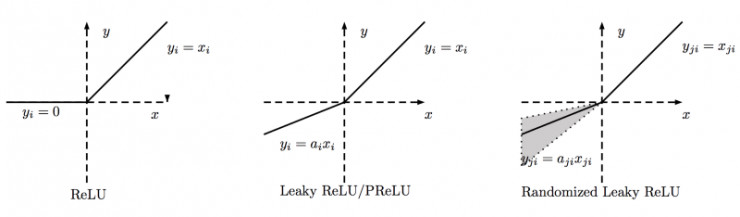PReLU中的ai是根据数据变化的；

Leaky ReLU中的ai是固定的；

RReLU中的aji是一个在一个给定的范围内随机抽取的值，这个值在测试环节就会固定下来。

ELU激活函数

函数定义：

函数图像如下：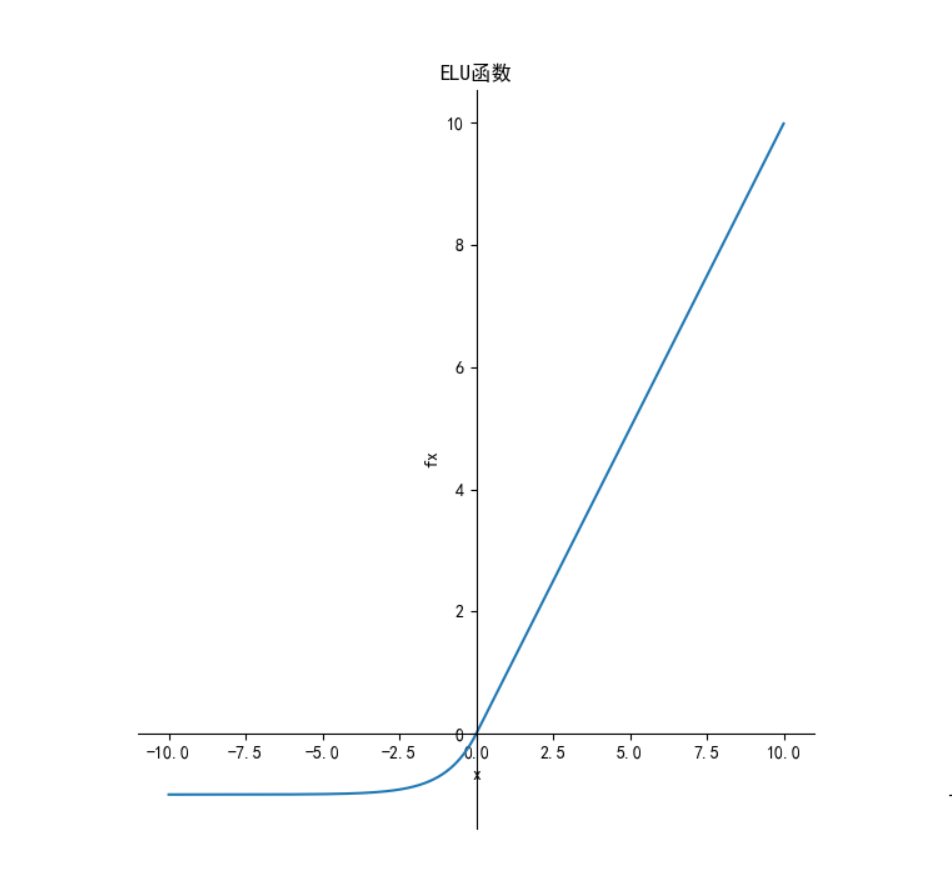导数：

函数图像如下：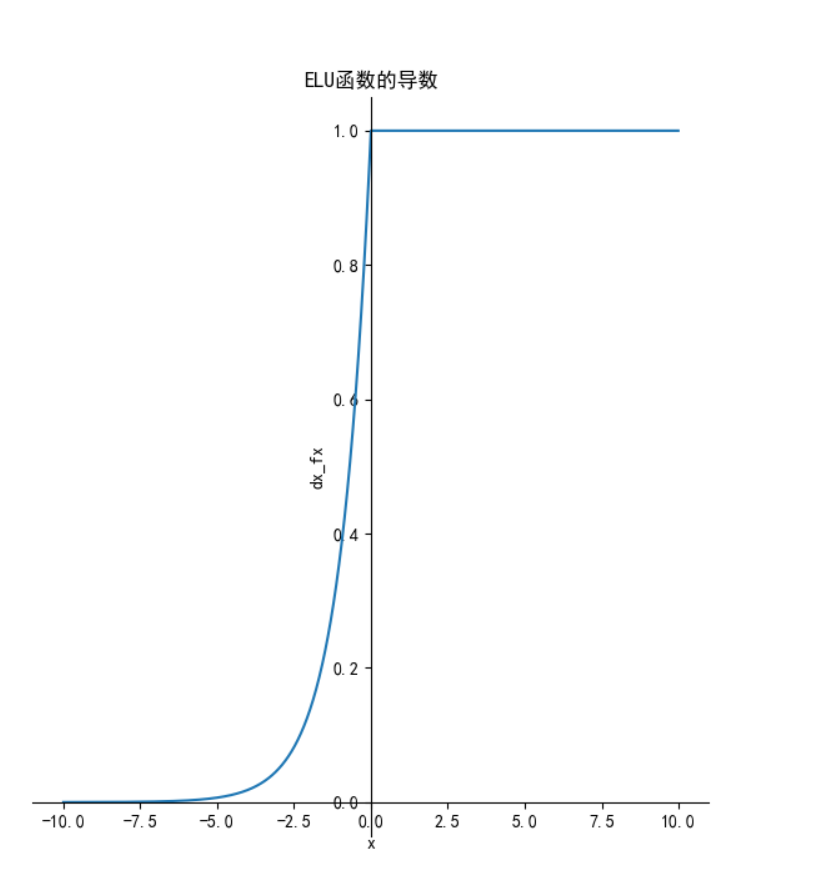特点：

融合了sigmoid和ReLU，左侧具有软饱和性，右侧无饱和性。
右侧线性部分使得ELU能够缓解梯度消失，而左侧软饱能够让ELU对输入变化或噪声更鲁棒。
ELU的输出均值接近于零，所以收敛速度更快。
在 ImageNet上，不加 Batch Normalization 30 层以上的 ReLU 网络会无法收敛，PReLU网络在MSRA的Fan-in （caffe ）初始化下会发散，而 ELU 网络在Fan-in/Fan-out下都能收敛。
函数及导数代码：

from matplotlib import pyplot as plt
import numpy as np

# 解决中文显示问题
plt.rcParams['font.sans-serif'] = ['SimHei']
plt.rcParams['axes.unicode_minus'] = False
def ELU(x):
"""ELU函数"""
return np.where(x<0,np.exp(x)-1,x)

def dx_ELU(x):
"""ELU函数的导数"""
return np.where(x < 0, np.exp(x), 1)

# ---------------------------------------------

if __name__ == '__main__':
x = np.arange(-10, 10, 0.01)
fx = ELU(x)
dx_fx = dx_ELU(x)
plt.subplot(1, 2, 1)
ax = plt.gca()  # 得到图像的Axes对象
ax.spines['right'].set_color('none')  # 将图像右边的轴设为透明
ax.spines['top'].set_color('none')  # 将图像上面的轴设为透明
ax.xaxis.set_ticks_position('bottom')  # 将x轴刻度设在下面的坐标轴上
ax.yaxis.set_ticks_position('left')  # 将y轴刻度设在左边的坐标轴上
ax.spines['bottom'].set_position(('data', 0))  # 将两个坐标轴的位置设在数据点原点
ax.spines['left'].set_position(('data', 0))
plt.title('ELU函数')
plt.xlabel('x')
plt.ylabel('fx')
plt.plot(x, fx)
plt.subplot(1, 2, 2)
ax = plt.gca()  # 得到图像的Axes对象
ax.spines['right'].set_color('none')  # 将图像右边的轴设为透明
ax.spines['top'].set_color('none')  # 将图像上面的轴设为透明
ax.xaxis.set_ticks_position('bottom')  # 将x轴刻度设在下面的坐标轴上
ax.yaxis.set_ticks_position('left')  # 将y轴刻度设在左边的坐标轴上
ax.spines['bottom'].set_position(('data', 0))  # 将两个坐标轴的位置设在数据点原点
ax.spines['left'].set_position(('data', 0))
plt.title('ELU函数的导数')
plt.xlabel('x')
plt.ylabel('dx_fx')
plt.plot(x, dx_fx)
plt.show()



Mish激活函数

函数定义：

,函数图像如下：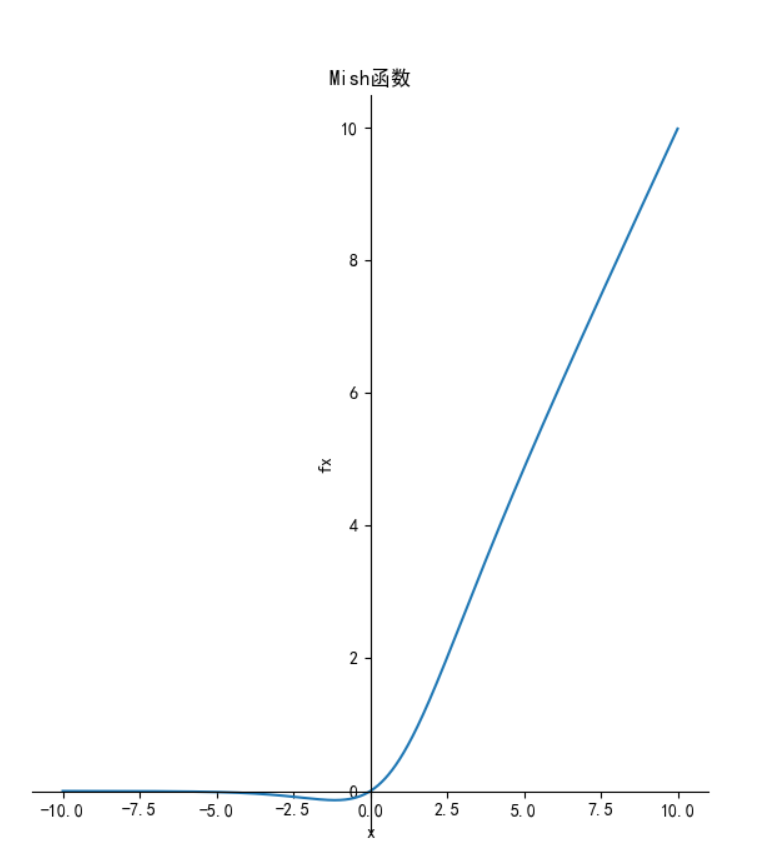导数：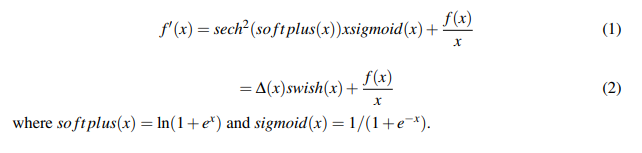函数图像如下：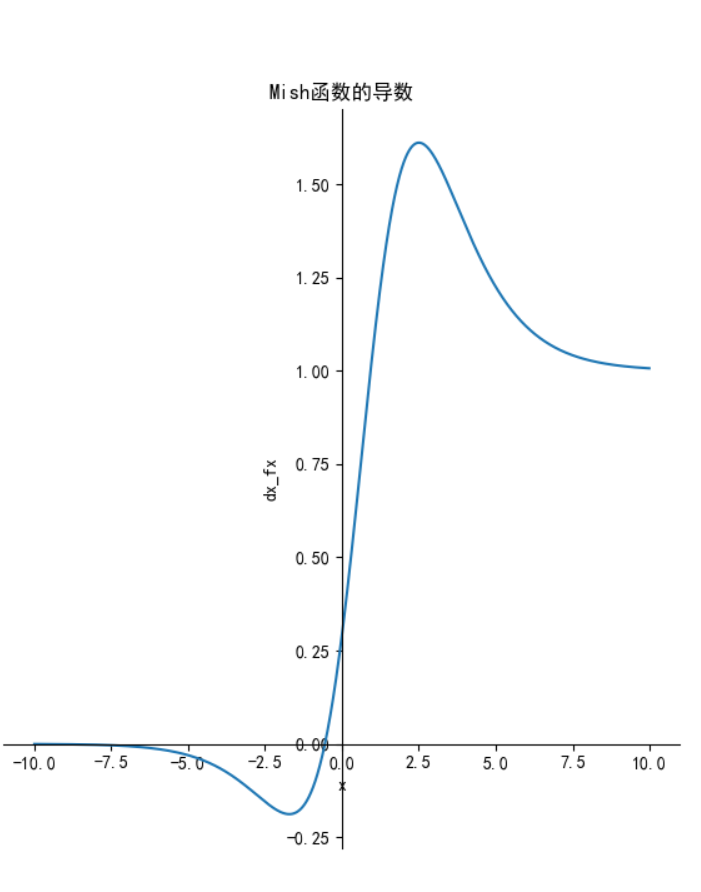特点：

特点：无上界(unbounded above)、有下界(bounded below)、平滑(smooth)和非单调(nonmonotonic)。
无上界：可以防止网络饱和，即梯度消失。
有下界：提升网络的正则化效果。
平滑：首先在0值点连续相比ReLU可以减少一些不可预料的问题，其次可以使网络更容易优化并且提高泛化性能。
非单调：可以使一些小的负输入也被保留为负输出，提高网络的可解释能力和梯度流
优点：平滑、非单调、上无界、有下界
缺点：引入了指数函数，增加了计算量

函数及导数代码：

from matplotlib import pyplot as plt
import numpy as np

# 解决中文显示问题
plt.rcParams['font.sans-serif'] = ['SimHei']
plt.rcParams['axes.unicode_minus'] = False
def sech(x):
"""sech函数"""
return 2 / (np.exp(x) + np.exp(-x))

def sigmoid(x):
"""sigmoid函数"""
return 1 / (1 + np.exp(-x))

def softplus(x):
"""softplus函数"""
return np.log10(1+np.exp(x))

def tanh(x):
"""tanh函数"""
return ((np.exp(x) - np.exp(-x)) / (np.exp(x) + np.exp(-x)))
if __name__ == '__main__':
x = np.arange(-10, 10, 0.01)
fx = x * tanh(softplus(x))
dx_fx = sech(softplus(x))*sech(softplus(x))*x*sigmoid(x)+fx/x
plt.subplot(1, 2, 1)
ax = plt.gca()  # 得到图像的Axes对象
ax.spines['right'].set_color('none')  # 将图像右边的轴设为透明
ax.spines['top'].set_color('none')  # 将图像上面的轴设为透明
ax.xaxis.set_ticks_position('bottom')  # 将x轴刻度设在下面的坐标轴上
ax.yaxis.set_ticks_position('left')  # 将y轴刻度设在左边的坐标轴上
ax.spines['bottom'].set_position(('data', 0))  # 将两个坐标轴的位置设在数据点原点
ax.spines['left'].set_position(('data', 0))
plt.title('Mish函数')
plt.xlabel('x')
plt.ylabel('fx')
plt.plot(x, fx)
plt.subplot(1, 2, 2)
ax = plt.gca()  # 得到图像的Axes对象
ax.spines['right'].set_color('none')  # 将图像右边的轴设为透明
ax.spines['top'].set_color('none')  # 将图像上面的轴设为透明
ax.xaxis.set_ticks_position('bottom')  # 将x轴刻度设在下面的坐标轴上
ax.yaxis.set_ticks_position('left')  # 将y轴刻度设在左边的坐标轴上
ax.spines['bottom'].set_position(('data', 0))  # 将两个坐标轴的位置设在数据点原点
ax.spines['left'].set_position(('data', 0))
plt.title('Mish函数的导数')
plt.xlabel('x')
plt.ylabel('dx_fx')
plt.plot(x, dx_fx)
plt.show()



Swish 激活函数

函数定义为：

，其函数图像如下：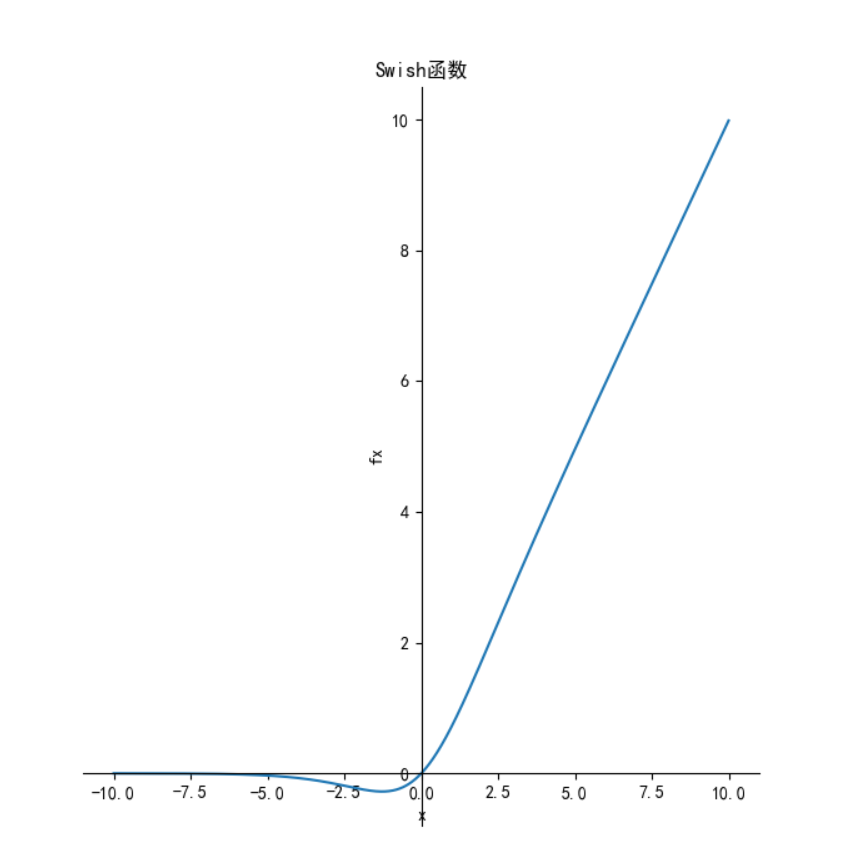其导数：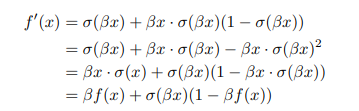函数图像如下：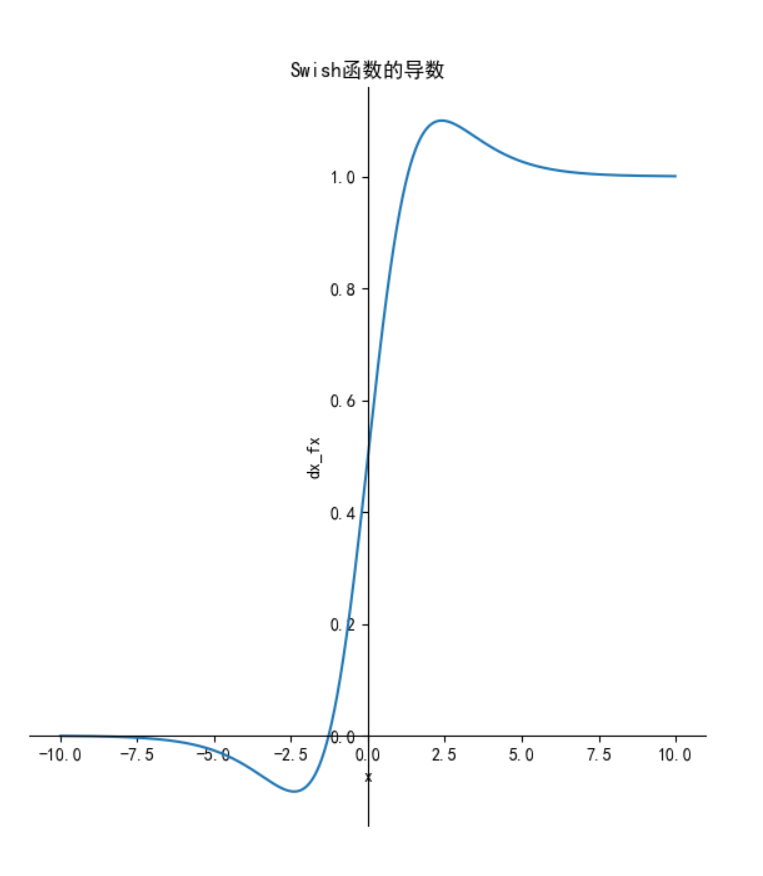特点：

特点：Swish 具备无上界有下界、平滑、非单调的特性。
优点：ReLU有无上界和有下界的特点，而Swish相比ReLU又增加了平滑和非单调的特点，这使得其在ImageNet上的效果更好。
缺点：引入了指数函数，增加了计算量

函数及导数代码：

from matplotlib import pyplot as plt
import numpy as np

# 解决中文显示问题
plt.rcParams['font.sans-serif'] = ['SimHei']
plt.rcParams['axes.unicode_minus'] = False
def sech(x):
"""sech函数"""
return 2 / (np.exp(x) + np.exp(-x))

def sigmoid(x):
"""sigmoid函数"""
return 1 / (1 + np.exp(-x))

def s(x):
"""sigmoid函数"""
return 1 / (1 + np.exp(-b*x))

if __name__ == '__main__':
x = np.arange(-10, 10, 0.01)
b = 1
fx = x / (1 + np.exp(-b * x))
dx_fx = b * fx + s(x) * (1 - b * fx)
plt.subplot(1, 2, 1)
ax = plt.gca()  # 得到图像的Axes对象
ax.spines['right'].set_color('none')  # 将图像右边的轴设为透明
ax.spines['top'].set_color('none')  # 将图像上面的轴设为透明
ax.xaxis.set_ticks_position('bottom')  # 将x轴刻度设在下面的坐标轴上
ax.yaxis.set_ticks_position('left')  # 将y轴刻度设在左边的坐标轴上
ax.spines['bottom'].set_position(('data', 0))  # 将两个坐标轴的位置设在数据点原点
ax.spines['left'].set_position(('data', 0))
plt.title('Swish函数')
plt.xlabel('x')
plt.ylabel('fx')
plt.plot(x, fx)
plt.subplot(1, 2, 2)
ax = plt.gca()  # 得到图像的Axes对象
ax.spines['right'].set_color('none')  # 将图像右边的轴设为透明
ax.spines['top'].set_color('none')  # 将图像上面的轴设为透明
ax.xaxis.set_ticks_position('bottom')  # 将x轴刻度设在下面的坐标轴上
ax.yaxis.set_ticks_position('left')  # 将y轴刻度设在左边的坐标轴上
ax.spines['bottom'].set_position(('data', 0))  # 将两个坐标轴的位置设在数据点原点
ax.spines['left'].set_position(('data', 0))
plt.title('Swish函数的导数')
plt.xlabel('x')
plt.ylabel('dx_fx')
plt.plot(x, dx_fx)
plt.show()



SiLU激活函数

函数定义为：

,其函数图形如下：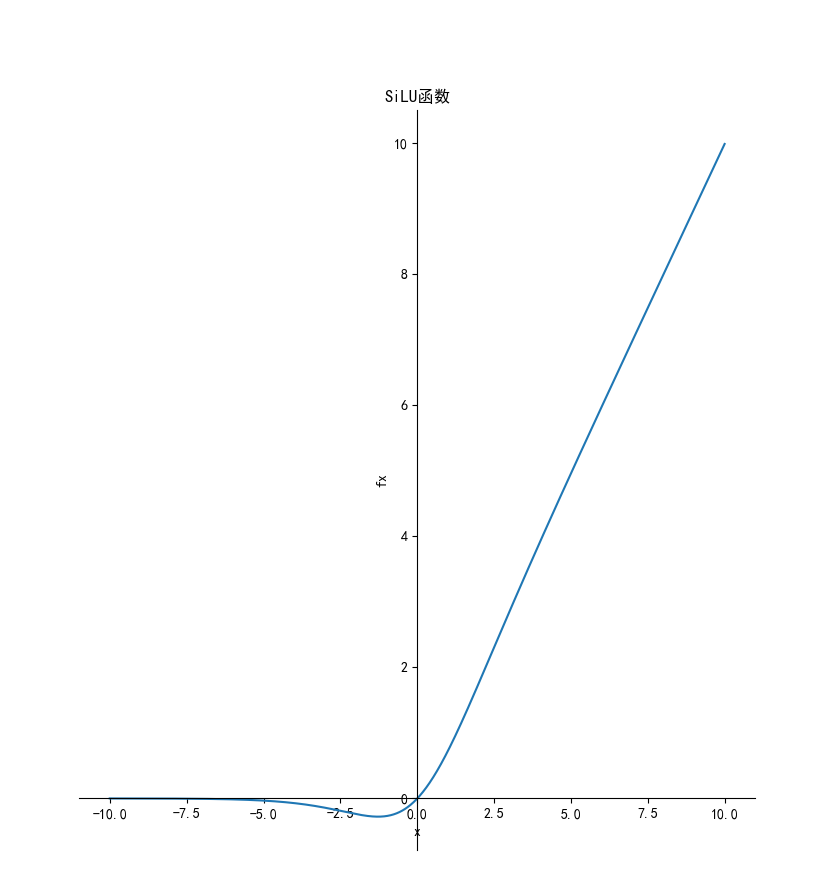导数为：

,其函数图像如下：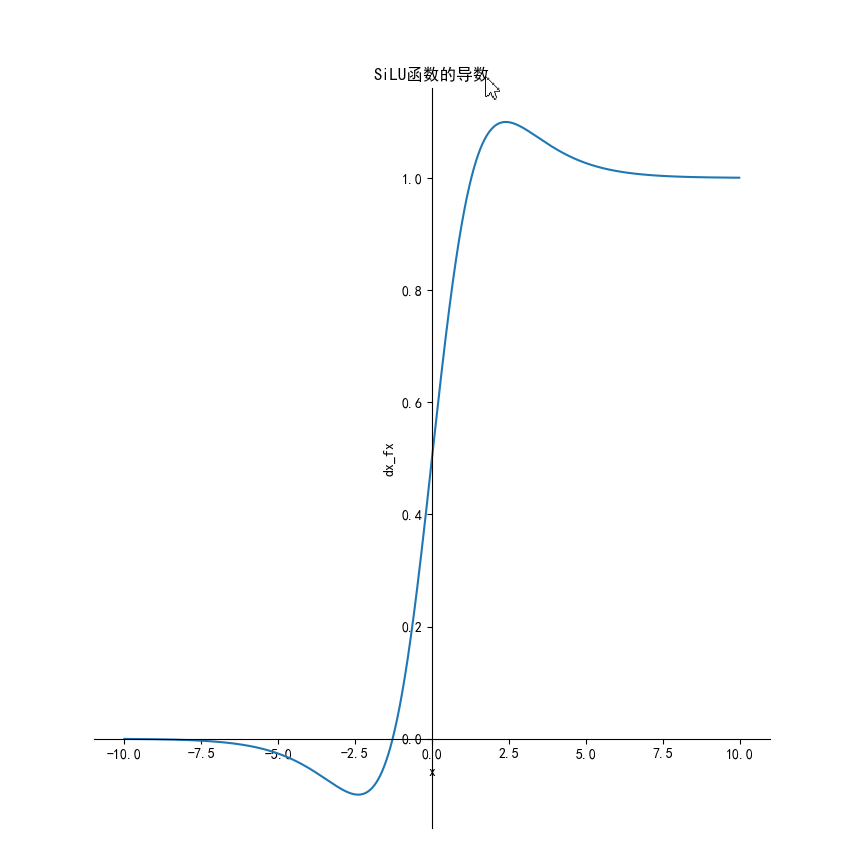函数及导数代码：

from matplotlib import pyplot as plt
import numpy as np

# 解决中文显示问题
plt.rcParams['font.sans-serif'] = ['SimHei']
plt.rcParams['axes.unicode_minus'] = False
def sigmoid(x):
y = 1 / (1 + np.exp(-x))
return y
def silu(x):
return x*sigmoid(x)
def dx_silu(x):
return silu(x)+sigmoid(x)*(1-silu(x))

if __name__ == '__main__':
x = np.arange(-10, 10, 0.01)
b = 1
fx = silu(x)
dx=dx_silu(x)
plt.subplot(1, 2, 1)
ax = plt.gca()  # 得到图像的Axes对象
ax.spines['right'].set_color('none')  # 将图像右边的轴设为透明
ax.spines['top'].set_color('none')  # 将图像上面的轴设为透明
ax.xaxis.set_ticks_position('bottom')  # 将x轴刻度设在下面的坐标轴上
ax.yaxis.set_ticks_position('left')  # 将y轴刻度设在左边的坐标轴上
ax.spines['bottom'].set_position(('data', 0))  # 将两个坐标轴的位置设在数据点原点
ax.spines['left'].set_position(('data', 0))
plt.title('SiLU函数')
plt.xlabel('x')
plt.ylabel('fx')
plt.plot(x, fx)
plt.subplot(1, 2, 2)
ax = plt.gca()  # 得到图像的Axes对象
ax.spines['right'].set_color('none')  # 将图像右边的轴设为透明
ax.spines['top'].set_color('none')  # 将图像上面的轴设为透明
ax.xaxis.set_ticks_position('bottom')  # 将x轴刻度设在下面的坐标轴上
ax.yaxis.set_ticks_position('left')  # 将y轴刻度设在左边的坐标轴上
ax.spines['bottom'].set_position(('data', 0))  # 将两个坐标轴的位置设在数据点原点
ax.spines['left'].set_position(('data', 0))
plt.title('SiLU函数的导数')
plt.xlabel('x')
plt.ylabel('dx_fx')
plt.plot(x, dx)
plt.show()




展开全文深度学习 机器学习 神经网络 人工智能
• 激活函数 关于激活函数的定义，该论文的作者有提到，激活函数的定义 如果没有激活函数，那么该网络仅能够表达线性映射，此时即便有再多的隐藏层，其整个网络跟单层神经网络也是等价的。因此也可以认为，只有...
一 激活函数
关于激活函数的定义，该论文的作者有提到，激活函数的定义
如果没有激活函数，那么该网络仅能够表达线性映射，此时即便有再多的隐藏层，其整个网络跟单层神经网络也是等价的。因此也可以认为，只有加入了激活函数之后，深度神经网络才具备了分层的非线性映射学习能力。激活函数不是真的要去激活什么。在神经网络中，激活函数的作用是能够给神经网络加入一些非线性因素，使得神经网络可以更好地解决较为复杂的问题。

可微性： 当优化方法是基于梯度的时候，这个性质是必须的。
单调性： 当激活函数是单调的时候，单层网络能够保证是凸函数。
输出值的范围： 当激活函数输出值是 有限 的时候，基于梯度的优化方法会更加 稳定，因为特征的表示受有限权值的影响更显著;当激活函数的输出是 无限 的时候，模型的训练会更加高效，不过在这种情况小，一般需要更小的learning rate

从目前来看，常见的激活函数多是分段线性和具有指数形状的非线性函数
注意：
激活函数中存在的一些概念：左右饱和，硬饱和，软饱和。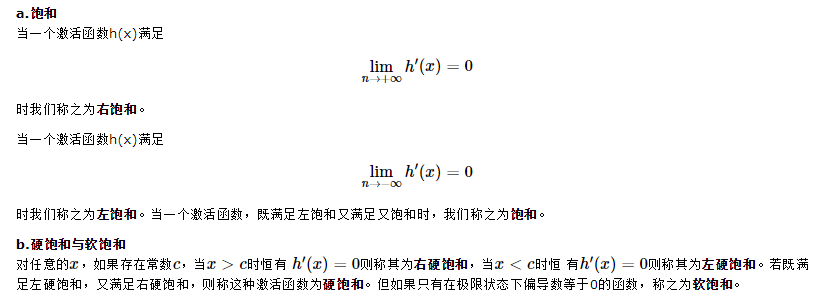以下介绍常见的激活函数：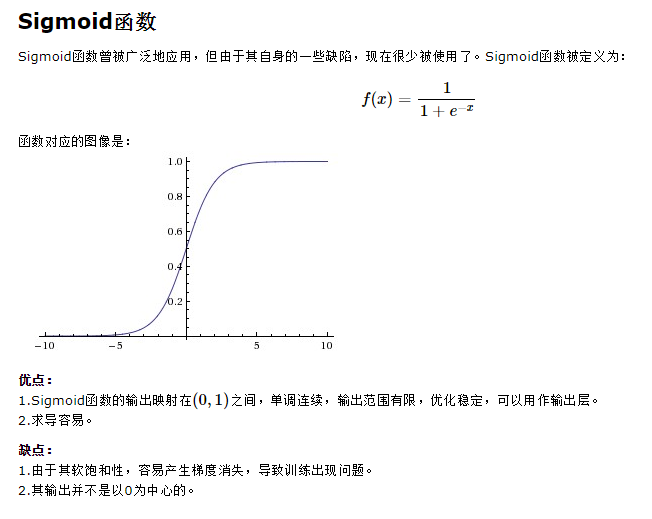sigmoid 是使用范围最广的一类激活函数，具有指数函数形状，它在物理意义上最为接近生物神经元。此外，(0, 1) 的输出还可以被表示作概率，或用于输入的归一化，代表性的如Sigmoid交叉熵损失函数。
然而，sigmoid也有其自身的缺陷，最明显的就是饱和性。从上图可以看到，其两侧导数逐渐趋近于0 。
sigmoid 的软饱和性，使得深度神经网络在二三十年里一直难以有效的训练，是阻碍神经网络发展的重要原因。具体来说，由于在后向传递过程中，sigmoid向下传导的梯度包含了一个 f′(x) 因子（sigmoid关于输入的导数），因此一旦输入落入饱和区，f′(x) 就会变得接近于0，导致了向底层传递的梯度也变得非常小。此时，网络参数很难得到有效训练。这种现象被称为梯度消失。一般来说， sigmoid 网络在 5 层之内就会产生梯度消失现象。
此外，sigmoid函数的输出均大于0，使得输出不是0均值，这称为偏移现象，这会导致后一层的神经元将得到上一层输出的非0均值的信号作为输入。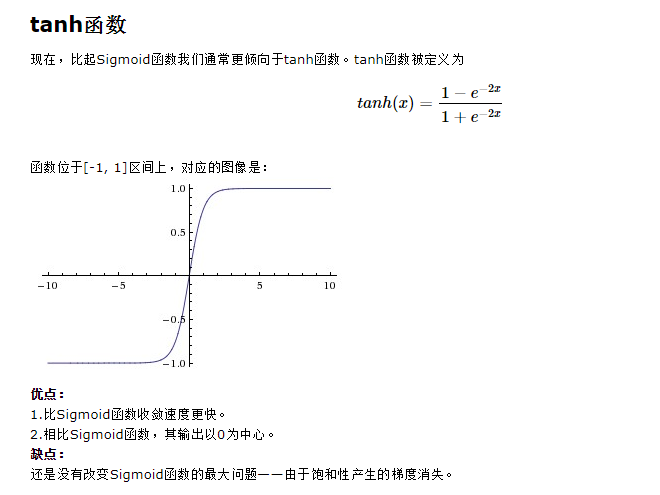tanh也是一种非常常见的激活函数。与sigmoid相比，它的输出均值是0，使得其收敛速度要比sigmoid快，减少迭代次数。然而，从途中可以看出，tanh一样具有软饱和性，从而造成梯度消失。

ReLU是最近几年非常受欢迎的激活函数。被定义为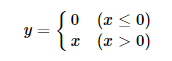对应的图像是：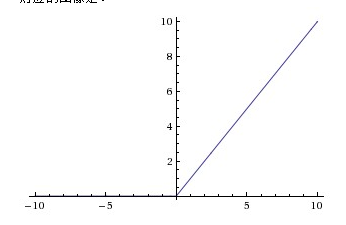可以看到，当x<0时，ReLU硬饱和，而当x>0时，则不存在饱和问题。所以，ReLU 能够在x>0时保持梯度不衰减，从而缓解梯度消失问题。这让我们能够直接以监督的方式训练深度神经网络，而无需依赖无监督的逐层预训练。
然而，随着训练的推进，部分输入会落入硬饱和区，导致对应权重无法更新。这种现象被称为“神经元死亡”。与sigmoid类似，ReLU的输出均值也大于0，偏移现象和 神经元死亡会共同影响网络的收敛性。
但是除了ReLU本身的之外，TensorFlow还提供了一些相关的函数，比如定义为min(max(features, 0), 6)的tf.nn.relu6(features, name=None)；或是CReLU，即tf.nn.crelu(features, name=None)。其中(CReLU部分可以参考这篇论文)。 优点：
1.相比起Sigmoid和tanh，ReLU(e.g. a factor of 6 in Krizhevsky et al.)在SGD中能够快速收敛。例如在下图的实验中，在一个四层的卷积神经网络中，实线代表了ReLU，虚线代表了tanh，ReLU比起tanh更快地到达了错误率0.25处。据称，这是因为它线性、非饱和的形式。
2.Sigmoid和tanh涉及了很多很expensive的操作（比如指数），ReLU可以更加简单的实现。
3.有效缓解了梯度消失的问题。
4.在没有无监督预训练的时候也能有较好的表现。
5.提供了神经网络的稀疏表达能力。 缺点：
随着训练的进行，可能会出现神经元死亡，权重无法更新的情况。如果发生这种情况，那么流经神经元的梯度从这一点开始将永远是0。也就是说，ReLU神经元在训练中不可逆地死亡了。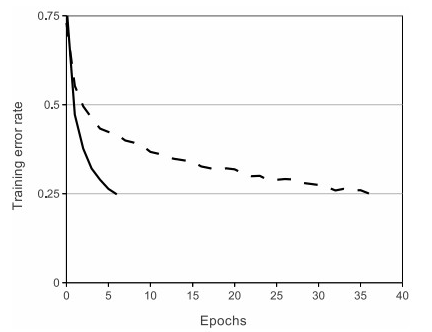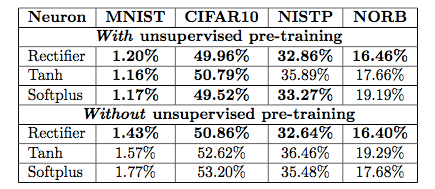针对在x<0的硬饱和问题，我们对ReLU做出相应的改进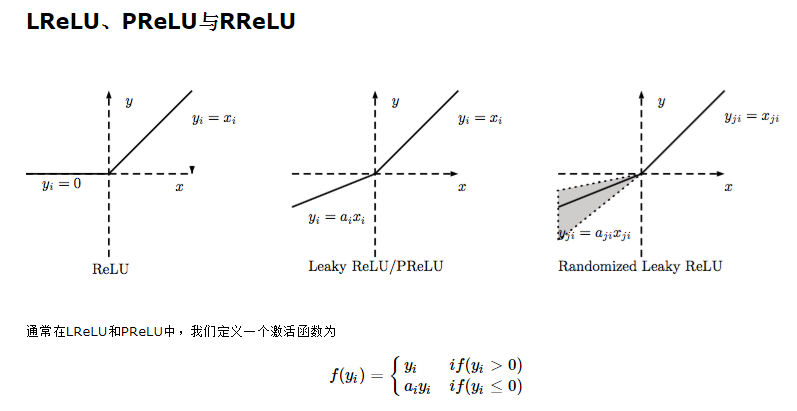当ai比较小而且固定的时候，我们称之为LReLU。LReLU最初的目的是为了避免梯度消失。但在一些实验中，我们发现LReLU对准确率并没有太大的影响。很多时候，当我们想要应用LReLU时，我们必须要非常小心谨慎地重复训练，选取出合适的a，LReLU的表现出的结果才比ReLU好。因此有人提出了一种自适应地从数据中学习参数的PReLU。 PReLU是LReLU的改进，可以自适应地从数据中学习参数。PReLU具有收敛速度快、错误率低的特点。PReLU可以用于反向传播的训练，可以与其他层同时优化。
在论文Delving Deep into Rectifiers: Surpassing Human-Level Performance on ImageNet Classification中，作者就对比了PReLU和ReLU在ImageNet model A的训练效果。值得一提的是，在tflearn中有现成的LReLU和PReLU可以直接用。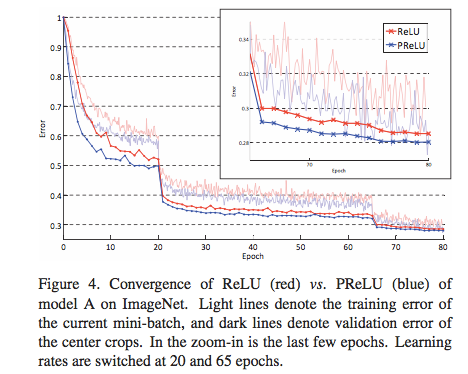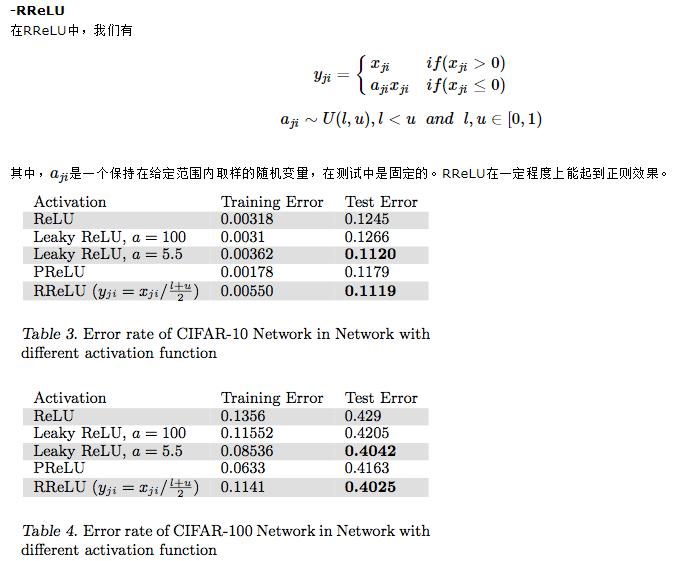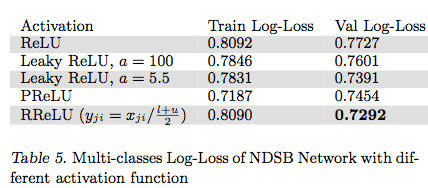在论文Empirical Evaluation of Rectified Activations in Convolution Network中，作者对比了RReLU、LReLU、PReLU、ReLU 在CIFAR-10、CIFAR-100、NDSB网络中的效果。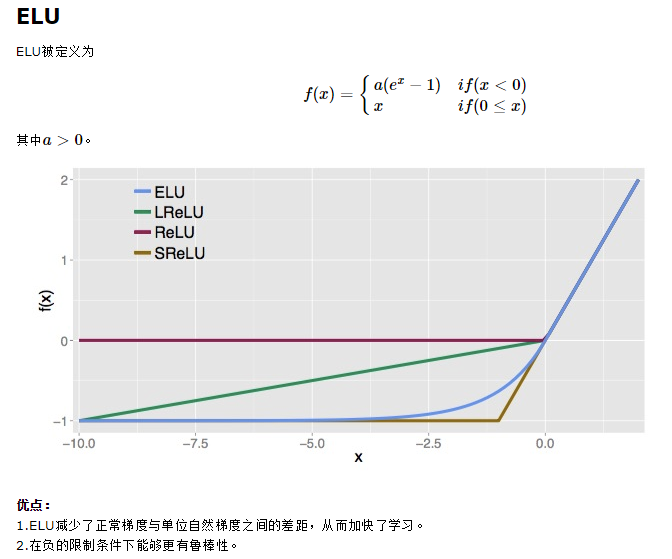融合了sigmoid和ReLU，左侧具有软饱和性，右侧无饱和性。右侧线性部分使得ELU能够缓解梯度消失，而左侧软饱能够让ELU对输入变化或噪声更鲁棒。ELU的输出均值接近于零，所以收敛速度更快。在 ImageNet上，不加 Batch Normalization 30 层以上的 ReLU 网络会无法收敛，PReLU网络在MSRA的Fan-in （caffe ）初始化下会发散，而 ELU 网络在Fan-in/Fan-out下都能收敛。 ELU的介绍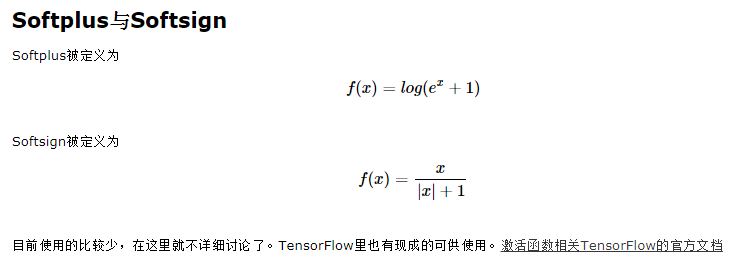Maxout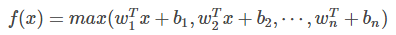损失函数部分有待后续补充。。。

主要参考了： 深度学习笔记(三)：激活函数和损失函数 浅谈深度学习中的激活函数
展开全文• CNN学习笔记：激活函数 激活函数 　激活函数又称非线性映射，顾名思义，激活函数的引入是为了增加整个网络的表达能力（即非线性）。若干线性操作层的堆叠仍然只能起到线性映射的作用，无法形成复杂的函数。常用的...
• Java代码中如何更改激活函数 更改前向传播forward()中卷积层激活函数。 更改输出层梯度计算公式 反向传播求激活函数微分 激活函数与标签值的修改 一、前言： 本文探讨在卷积神经网络结构中，如何去处理...
• 1、激活函数 更新W有两种方法：穷举法、梯度下降法 如何选择激活函数？ 通常来说，很少会把各种激活函数串起来在一个网络中使用。 如果使用 ReLU ，那么一定要小心设置学习率（learning rate ），预防出现过多...
• 激活函数 　使用一个神经网络时，需要决定使用哪种激活函数用隐藏层上，哪种用在输出节点上。 　在神经网路的前向传播中，这两步会使用到sigmoid函数。sigmoid函数在这里被称为激活函数。 sigmoid函数 　之前在...
• 对于二分类问题，可以直接使用sigmoid函数，输出层只需要有一个神经元就可以 对于二分类问题，如果使用softmax，就得使用两个神经元作为输出层，每个神经元代表一个类别 大于二的分类问题，不能使用sigmoid ...
• 为什么要使用激活函数？ 我们训练的神经网络模型，就是从输入到输出的一个神秘未知函数映射。我们并不知道具体的内容，只能尽可能无限的逼近它。 如果不使用激活函数，那么输出只能是线性的。 如果使用了非线性激活...
• 假设我们选择的激活函数是σ(z)σ(z)，隐藏层和输出层的输出值为aa，则对于下图的三层DNN,利用和感知机一样的思路，我们可以利用上一层的输出计算下一层的输出，也就是所谓的DNN前向传播算法。    ...
• 当用经典的 LeNet 进行 MNIST 识别训练时，我发现将 sigmoid 函数改为 ReLu，同时降低学习率（防止 dying neurons）并没能加快 SGD 收敛，反而使得 accuracy 的提升变得非常缓慢。所以这样看来，当 CNN 没有深到极易...
• 激活函数 为什么要用激活函数？ 如果不用激励函数，每一层输出都是上层输入的线性函数，无论神经网络有多少层，输出都是输入的线性组合。 如果使用的话，激活函数给神经元引入了非线性因素，使得神经网络可以任意...
• 深度学习基础理论-CNN激活函数激活函数（activation function）层又称非线性映射层，顾名思义，激活函数的引入为的是增加整个网络的表达能力（即非线性...
• CNN中的窗口理解 窗口大小，就是局部感知视野 图片的深度depth，也叫channel depth深度：图片是从几个方面进行描述的。...CNN中的激活函数 ReLU效果不好的话再考虑用Leaky ReLU Maxout不是纯粹的激活函数，但可以把...深度学习 计算机视觉
• 激活函数概述 identiti函数 sigmoid函数 relu函数 leaky_relu elu softmax tanh tanh p1m2 源码阅读 参考激活函数概述神经网络都有各个层组成，在不同的层中，用到不同的激活函数。在看layer之前，先了解一下定义的...神经网络 c++11 机器学习
• 卷积神经网络中激活函数，理论上可以是线性函数：例 但是在模型中一般选用非线性的激活函数。 (1)sigmoid函数： (2)双曲正切函数： (3)硬限幅函数 (4)斜面函数 （5）修正线性单元函数（ReLU） （6）...神经网络
• 提纲： 1、感受野 2、优化函数 sgd adam rmspop。。。。 3、activation ReLU LeakyReLU 等 4、padding计算方式，卷积后尺寸
• import tensorflow as tf ...学习 relu激活函数 和 dropout使用 """ tf.set_random_seed(43) def activation_func(): with tf.Graph().as_default(): hidden_layer_weights = tf.truncated_normal(shape=[4,...
• Mish激活函数：f(x)=x⋅tanh⁡(softplus(x))=x⋅tanh⁡(ln⁡(1+ex))f(x)=x\cdot \tanh(softplus(x))=x\cdot \tanh(\ln(1+e^x))f(x)=x⋅tanh(softplus(x))=x⋅tanh(ln(1+ex)) relu激活函数：f(x)=max{0,x}f(x)=max\{0...
• 8.1 Sigmoid 型函数 σ(x)=11+e(−x)σ(x)=11+e(−x) \sigma(x)=\frac{1}{1+e^{(-x)}} sigmoid 将输出响应的值域压缩到[0,1]之间 但是，两端大于5，小于-5的区域，梯度接近0，带来梯度的“饱和效应” 反向传播...
• 这里是波波给大家带来的CNN卷积神经网络入门讲解 不定期我将给大家带来绝对原创，脑洞大开，幽默风趣的深度学习知识点入门讲解 希望大家多多支持，多多关注 微信公众号：follow_bobo 知乎号：蒋竺波 ...
• 池化是使用某一位置的相邻输出的总体统计特征代替网络在该位置的输出，其好处是当输入数据做出少量平移时，经过池化函数后的大多数输出还能保持不变。比如：当识别一张图像是否是人脸时，我们需要知道人脸左边有一只...
• 可能仅仅适应于全连接，将全连接种的torch.sin换成mish或者swish的时候，反而不如relu 链接是:https://github.com/WANG-KX/SIREN-2D(不是) Mish(前两个分别是relu和 relu+PE) Swish(前两个分别是r深度学习 pytorch
• CNN/RNN各种模型激活函数总结 0.Sigmoid： Sigmoid函数是早期非常经典的激活函数，输出值在 [0,1] 之间，当输入值为非常大负数时，值为0，当输入值非常大正数时，值为1。Sigmoid非常经典，但是现在它以不太受欢迎，...
• 1，激活函数有什么作用，常用的的激活函数 2，怎么解决梯度消失问题 BN Relu 使用 ReLU、LReLU、ELU、maxout 等激活函数 sigmoid函数的梯度随着x的增大或减小和消失，而ReLU不会。 使用批规范化 通过规范化操作将...
• https://arxiv.org/pdf/1912.10946.pdf 本文是sigmoid函数的改进，主要在resnet18，resnet50模型上验证了其有效性。 ...
• 研究了现阶段的激活函数，将激活函数大致分为S系激活函数和ReLU系激活函数，从不同激活函数的功能特点和存在的饱和性、零点对称和梯度消失及梯度爆炸的现象进行研究分析，针对Sigmoid，Tanh，ReL，P-ReLU，L-ReLU等......